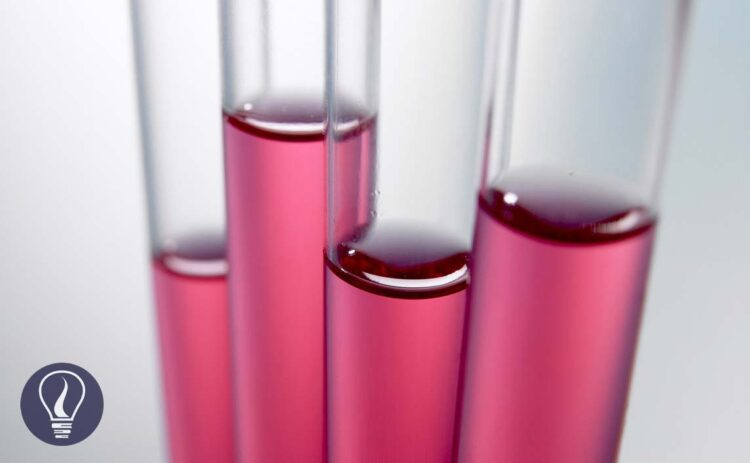More results...

Generic selectors
Exact matches only
Search in title
Search in content
Post Type Selectors

# How to Find the Concentration of a SolutionB.Sc (Hons, USJ) (Polymer Science and Technology, Chemistry, Physics)
Categories
Tags :
Last Updated On : January 20, 2023
Published Date : January 7, 2023

## How to find the concentration of a solution - Molarity (M) of a solution

Solutions are created by dissolving solutes in solvents. The concentration of a solution is the dissolved amount of moles of the solute, in a unit volume of the solution at a given temperature. If the dissolved amount of solute moles is high, the concentration of the solution would be high.

SI units of the amount of substance are mol, and the Volume is m-3. Therefore, the SI units of the concentration would be mol m-3. But generally, the concentration is expressed in mols per decimeter. (mol dm-3). Concentration is also known as molarity (M) and mol concentration.

Example 01

A glucose solution has been prepared by dissolving 5 mol of glucose in 2 dm-3 of water. Find the concentration of the solution.

## Normality (N)

Normality is the number of mols of equivalents in the solute in a unit volume of a solvent.

The term equivalent is defined as the reactive capacity of a chemical species. The equivalent can be either ions or electrons.

Example 02

In 5 dm-3 of an aqueous solution of H2SO4, there are 6 mols of H2SO4. Find the normality of the solution.

The number of Carbon-12 atoms in exactly 12.0g of Carbon -12 (126C) is known as the Avogadro constant. The value of the Avogadro constant is 6.022*1026

## Mole

Mole is the international unit for the “amount of substance”. If we take Avogadro number of any substance, it is a mole. As an example

• Avogadro number of atoms = 1 mol of atoms
• Avogadro number of molecules = 1 mol of molecules
• Avogadro number of ions = 1 mol of ions
• Avogadro number of electrons = 1 mol of electrons

### Relation between the mole and relative atomic mass

For each element, the number of grams in a mole equals the number of atomic mass units in the element's atomic mass. In other words, if we take the number of grams similar to the relative atomic mass unit of a particular element, there is one mole of that element in it. Since this is the mass of the one mole of an element, we can call it a “Molar mass”. Further, 1 mol of an element contains Avogadro number of atoms.

For example, if we take 15.9994g of oxygen (relative atomic mass unit of Oxygen = 15.9994), there is one mole of oxygen. And there is Avogadro's number of oxygen atoms.

If we take 180.156g of glucose, there is 1 mole of glucose molecules. And there is Avogadro's number of glucose molecules.

The relationship between mass, molar weight, and the mole can be expressed as follows.

Example 03

A sodium chloride solution has been prepared by dissolving 293.0g of NaCl in 2 dm-3 of water. Find the concentration of the solution. The molar mass of NaCl is 58.44 g/mol First, we should calculate the mols that have dissolved in the solution.

## Mol fraction (mole fraction)

In a mixture with multiple components, the ratio of the concentration of each component to the total moles in the mixture is called the mol fraction.

Let’s consider there is a mixture of components of A, B, and C. Number of the mols of each component are nA, nB, and nC respectively. Mol fraction of components A, B, and C can be calculated as follows.

The sum of each mole fraction of each component in the system is equal to 1. Mol fraction is a unitless quantity.

Example 04

In a gaseous system, there is 0.1 mol of CO2, 0.4 mol of O2, and 1.5 mol of N2. Find the mol fractions of each component in the system.

## Mass fraction

The ratio of the mass of a component to the total mass of the mixture is called 'mass fraction'.

We can get the mass percentage of components by multiplying the mass fraction by 100. In other words, the mass percentage is the number of the parts of the component in the 100 parts of the mixture. Therefore, the mass percentage is also known as the parts per hundred (pph). We can obtain parts per thousand (ppt), parts per million (ppm), parts per billion (ppb) so on by multiplying 103, 106, and 109 respectively.

Example 05

In 50g of a metal alloy, there is 75mg Ni. Find the mass fraction of Nickel.## References and Attributes

### Figures:

The cover image was created using an image by Steve Cross from Pixabay